# Important Simple Harmonic Motion Formulas For JEE Main and Advanced

If the restoring force/torque acting on the body in oscillatory motion is directly proportional to the displacement of the body and is always directed towards equilibrium position then the motion is called Simple Harmonic Motion (SHM). Find all the equations and formulas related to Simple Harmonic Motion in this page.

## Simple Harmonic Motion Formulas

1. General Equation of SHM

Displacement x =A sin(ωt + Φ)

Here (ωt + Φ) is the phase of the motion and Φ is the initial phase of the motion

2. Angular Frequency (ω)

ω = 2π/T = 2πf

T is the time period

f is the frequency

3. Frequency (f):

Number of oscillations completed in the unit time interval

f = 1/T = ω/2π

4. Time Period (T)

T = 2π/ω

5. Hooke’s law

Force (F) = -kx (for small elongation x)

k is the spring constant

6. Acceleration

a = -ω2x = -ω2 A sin(ωt + Φ)

7. Velocity

v = Acos(ωt+ Φ) =$\pm \omega \sqrt{A^{2}-x^{2}}$

Φ is the initial phase of motion

8. Potential Energy

U = ½ kx2 (as a function of x)

9. Kinetic Energy

K = ½ mω2(A2 – x2)

K = ½ k(A2 – x2)

10. Total Energy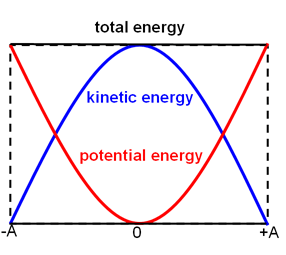E = U+K

E= ½ mω2A2

11. Simple Pendulum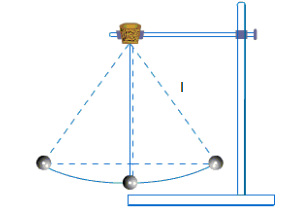$T=2\pi \sqrt{l/g}$

l is the length of the pendulum

12. Physical Pendulum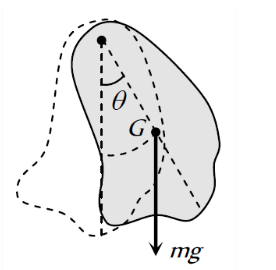$T=2\pi \sqrt{I/mgl}$

Here I= Icm + ml 2

l is the distance between the point of suspension and centre of mass.

13. Torsional Pendulum

$T=2\pi \sqrt{I/k}$

k is the torsional constant

14. Springs in parallel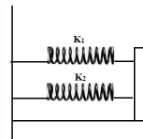Keq = k1+k2

15. Springs in series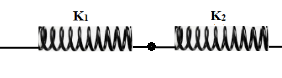1/keq = 1/k1 + 1/k2